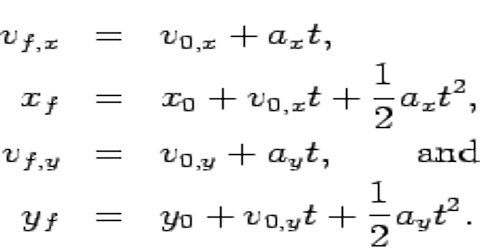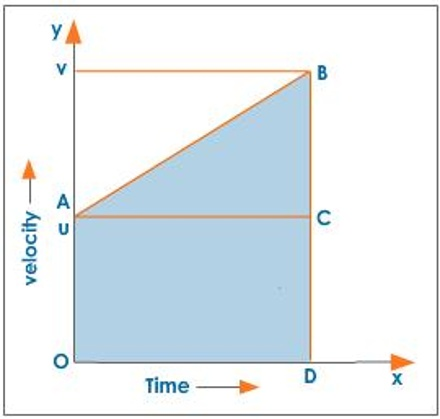# Third Equation of Dimensional Motion

Third Equation of Dimensional Motion

In one dimensional motion a body moves along a straight line. So quantities associated with motion, for example displacement, velocity, acceleration etc., have only one component (moving along X-axis will have X-component and Y and Z components will be zero). In deriving equations of linear motion we will consider that the body is moving along X-axis. In that case subscripts associated with different quantifies of motion may be omitted. Normally, vx will be represented by v and ax by a.Third Equation

Equation of motion relating position or displacement, acceleration and time: S =v0t + ½ st2.

or, x = x0 + vx0t + ½ axt2

If a body is moving with uniform acceleration its average velocity is equal to half of the sum of the initial velocity v0 and the final velocity v.

Thus, average velocity v = (v0 + v) / 2

But we know, v = v0 + at

So, average velocity, v = (v0 + v0 + at) / 2

= v0 + ½ at … … … (1)

Again we know average velocity,

v = s/t = displacement/time

or, s = vt … … … (2)

Now putting v from equation (1) to equation (2); we get:

S = (v0 + ½ at) t = v0t + ½ at2 … … … (3)

For one dimensional motion, say along X-axis, let the initial position at t = 0 is x0 and at time t = t. the position is x. So, the change of position, displacement, s = x – x0. Now, if initial velocity is vx0 and the final velocity v and the acceleration a, then from equation (3) we get;

S = x – x0 = vx0t + ½ axt2

or, x = x0 + vx0t + ½ axt2